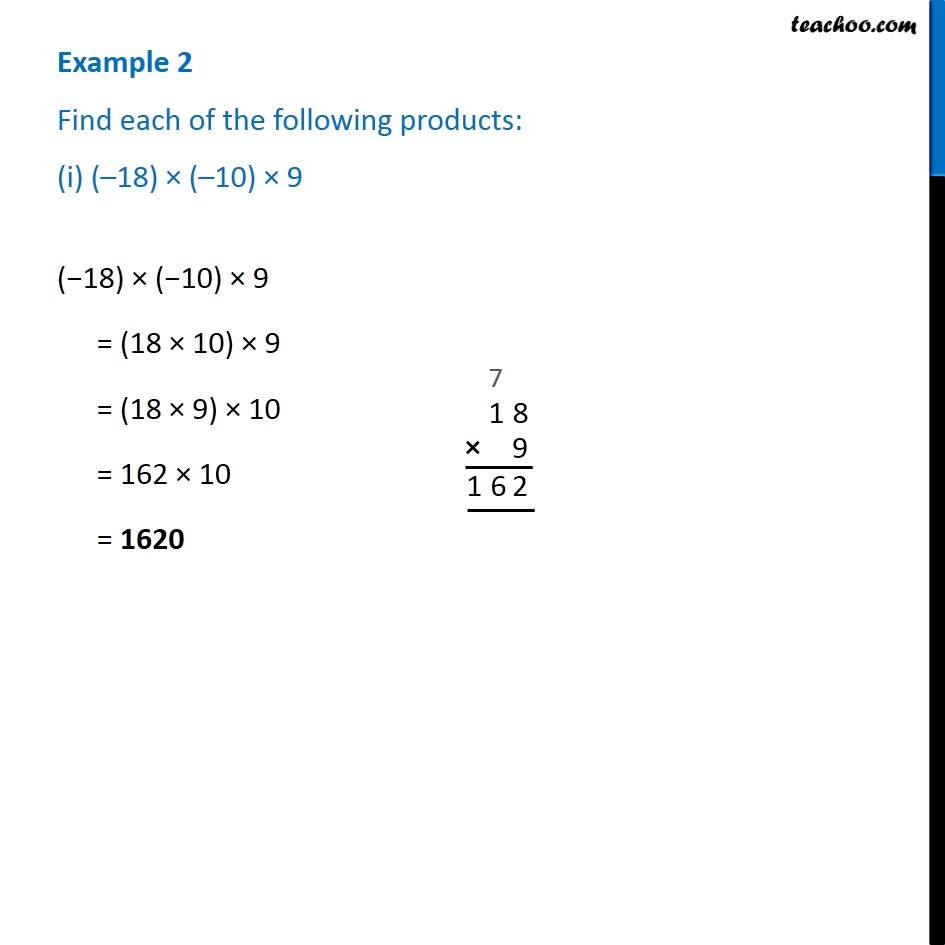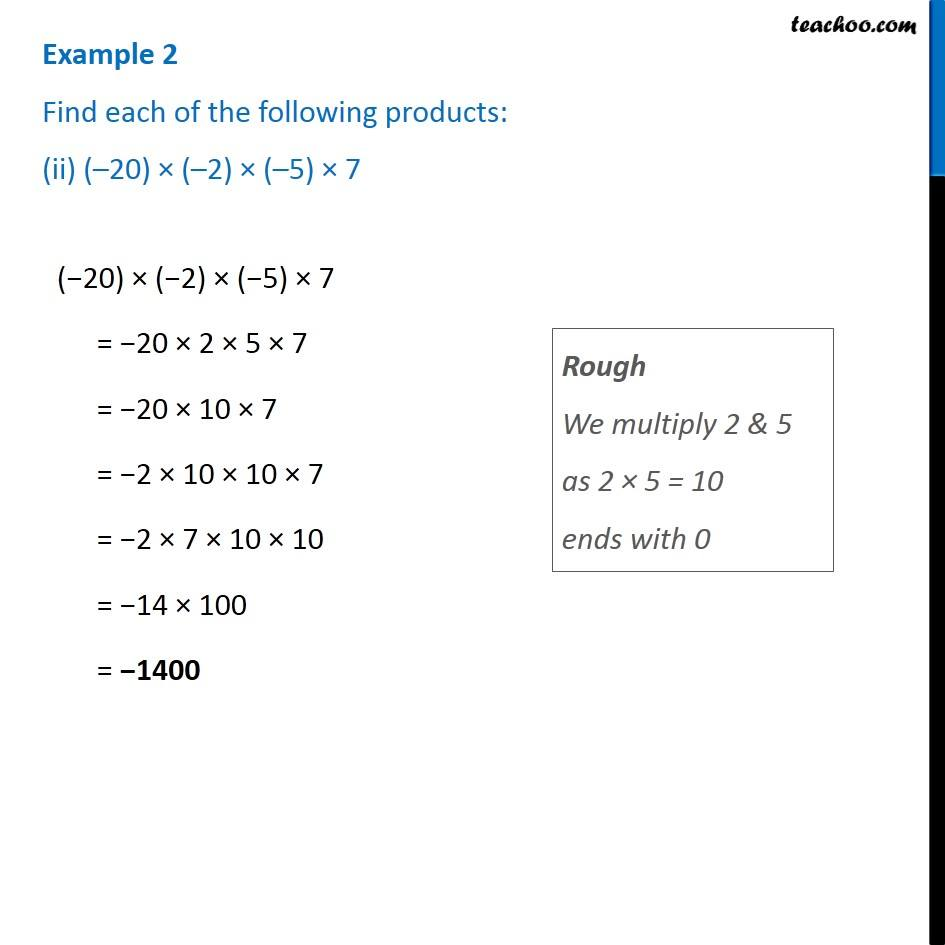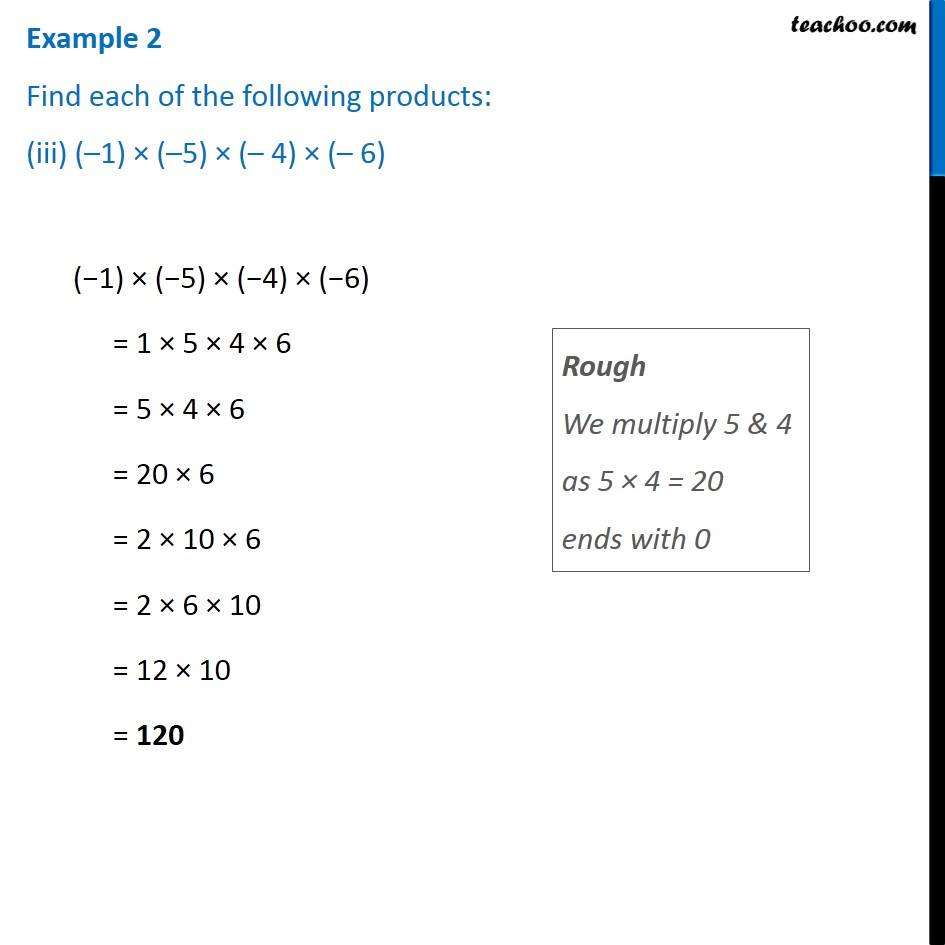Subscribe to our Youtube Channel - https://you.tube/teachoo

1. Chapter 1 Class 7 Integers
2. Serial order wise
3. Examples

Transcript

Example 2 Find each of the following products: (i) ( 18) ( 10) 9 ( 18) ( 10) 9 = (18 10) 9 = (18 9) 10 = 162 10 = 1620 Example 2 Find each of the following products: (ii) ( 20) ( 2) ( 5) 7 ( 20) ( 2) ( 5) 7 = 20 2 5 7 = 20 10 7 = 2 10 10 7 = 2 7 10 10 = 14 100 = 1400 Rough We multiply 2 & 5 as 2 5 = 10 ends with 0 Example 2 Find each of the following products: (iii) ( 1) ( 5) ( 4) ( 6) Rough We multiply 5 & 4 as 5 4 = 20 ends with 0 ( 1) ( 5) ( 4) ( 6) = 1 5 4 6 = 5 4 6 = 20 6 = 2 10 6 = 2 6 10 = 12 10 = 120

Examples

Chapter 1 Class 7 Integers
Serial order wise